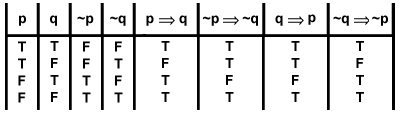# Is If X, then Y. Not Y. Therefore, not X. correct?

If X, then Y. Not Y. Therefore, not X.

I don't have the logical or mathematical sophistication to know if this is a correct conclusion or not.

Yes, this is Modus Tollens, a Hypothetical that reaches its conclusion by denying the consequent of a conditional statement: If X, then Y. Not Y. Therefore not X. It is deductively valid.

However, If X, then Y. Not X. Therefor not Y. This is deductively invalid. It is a fallacy called "Denying the Antecedent," a hypothetical that reaches its conclusion by denying the antecedent of a conditional statement.

• Hypothetical syllogism is not involved... See en.wikipedia.org/wiki/Hypothetical_syllogism Jun 15 '16 at 15:09
• You might want to add to this that the truth of Modus Tollens can easily be checked via a truth table. That's usually the starting point for people when they study logic for the first time, so that might be of some help.
– MM8
Jun 17 '16 at 13:28

The statement (¬Y)→(¬X) is called the contrapositive of the statement XY. A statement and its contrapositive are always logically equivalent.

All this can also nicely be shown by a truth table, like so: